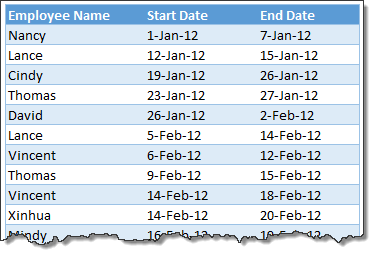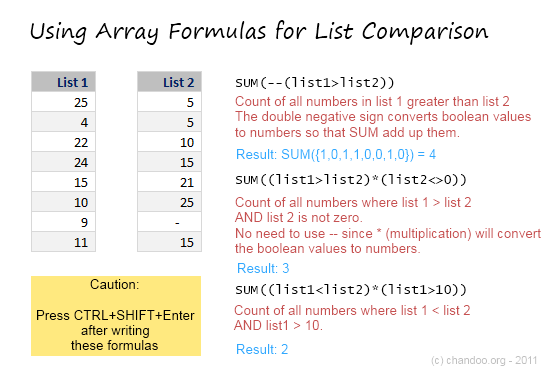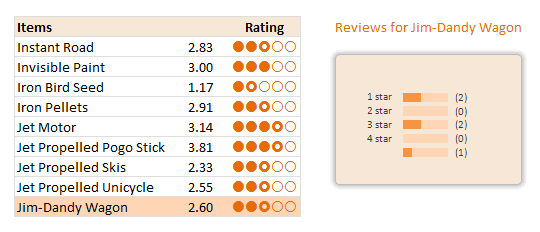# All articles with 'countifs' Tag

## Can you calculate vacation days in a period? [Homework]

Published on Jan 25, 2013 in Excel ChallengesIts Friday, that means time for another Excel challenge for you.

Calculate vacation days in a period:

Your mission, if you choose to accept it,

Step 2: Calculate number of vacations taken in a period. Specifically,

1) How many vacations are taken between start & end dates, assuming complete vacation should be inside the start & end date period?
2) How many vacations are taken such that at least one day of vacation is between start & end dates?
3) How many people took vacations? (if same person took multiple vacations, then count it as 1)

Continue »

## Comparing Lists of Values in Excel using Array Formulas

Published on Jun 14, 2011 in Excel Howtos, Learn ExcelLast week, we had a home work on Calculating Donation Summaries using Excel Formulas. This is a good case where array formulas can help us. So today, we will learn how we can use Array Formulas to compare lists of values and calculate summaries. Towards the end of this post, you can see a video that explains the solution to Donation Summary Calculation problem.

Continue »

## Show Details On-demand in Excel [Tutorial + Training Program]

Published on Apr 7, 2011 in Charts and Graphs, products, VBA MacrosYesterday, we have seen a beautiful example of how showing details (like distribution) on-demand can increase the effectiveness of your reports. Today, we will learn how to do the same in Excel.

Continue »

### Get FREE Excel & Power-BI Newsletter

One email per week with Excel and Power BI goodness. Join 100,000+ others and get it free.